# 漫画：什么是希尔排序？​————— 第二天 —————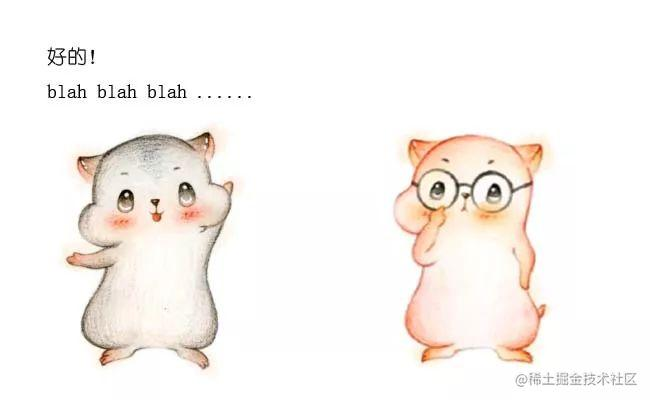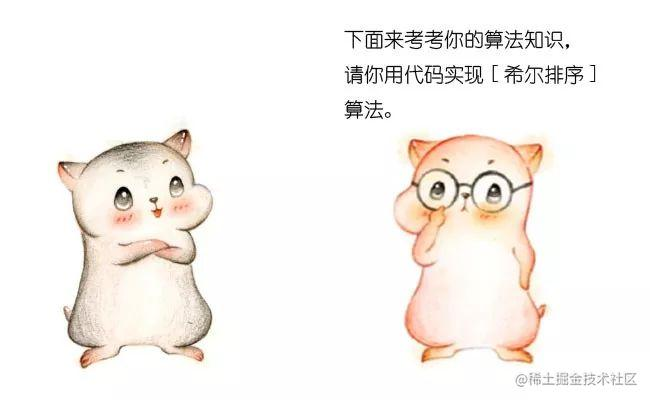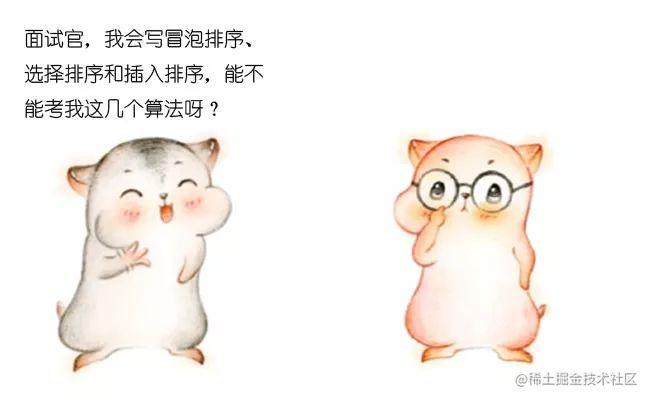————————————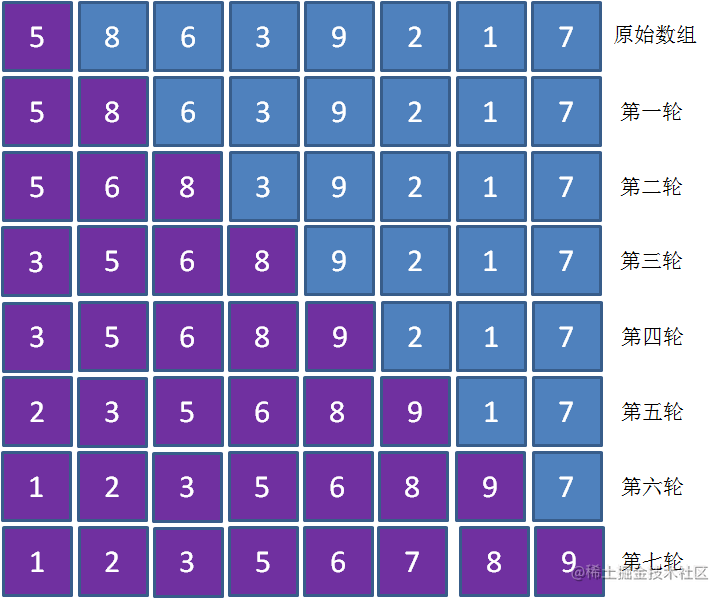1.在大多数元素已经有序的情况下，插入排序的工作量较小

2.在元素数量较少的情况下，插入排序的工作量较小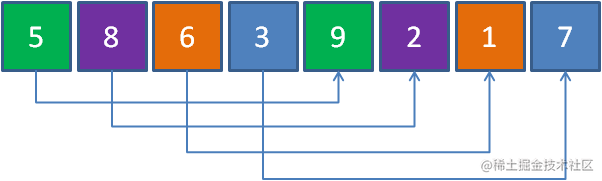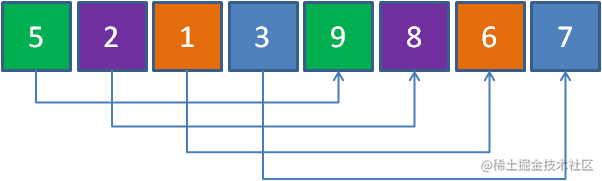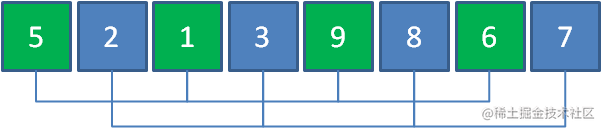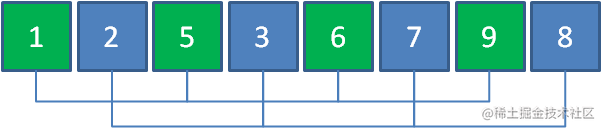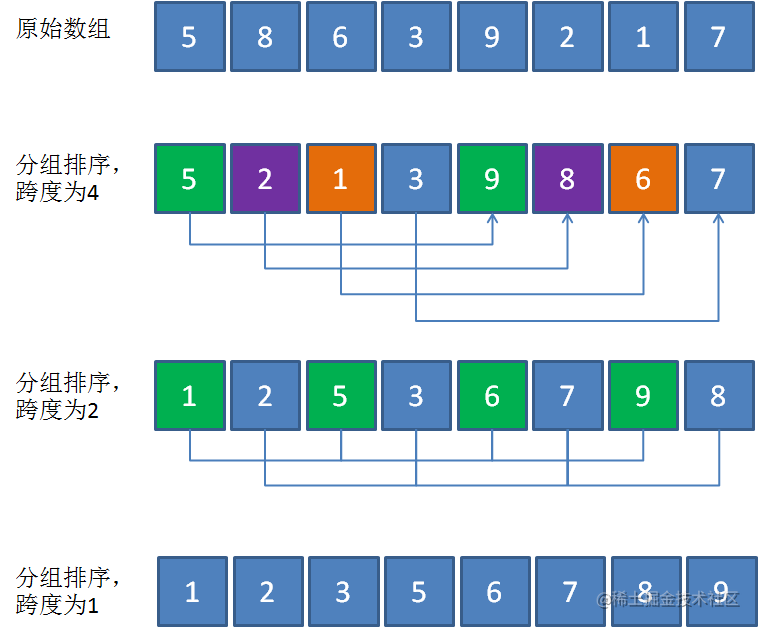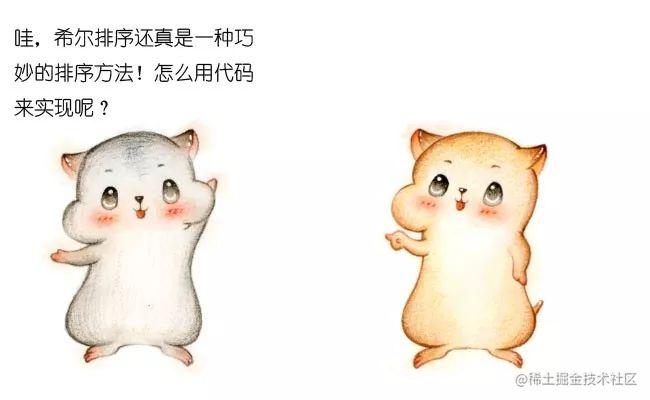``public static void sort(int [] array){    //希尔排序的增量    int d=array.length;    while(d>1) {        //使用希尔增量的方式，即每次折半        d=d/2;        for(int x=0;x<d;x++) {            for(int i=x+d;i<array.length;i=i+d) {                int temp=array[i];                int j;                for(j=i-d;j>=0&&array[j]>temp;j=j-d) {                    array[j+d]=array[j];                }                array[j+d]=temp;            }        }    }}public static void main(String [] args){    int[] array = {5,3,9,12,6,1,7,2,4,11,8,10};    sort(array);    System.out.println(Arrays.toString(array));}复制代码``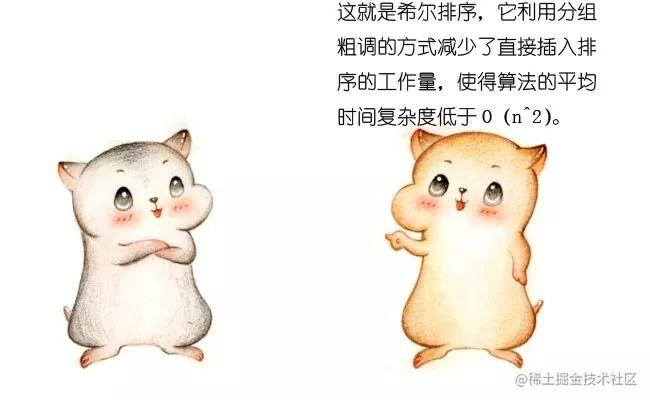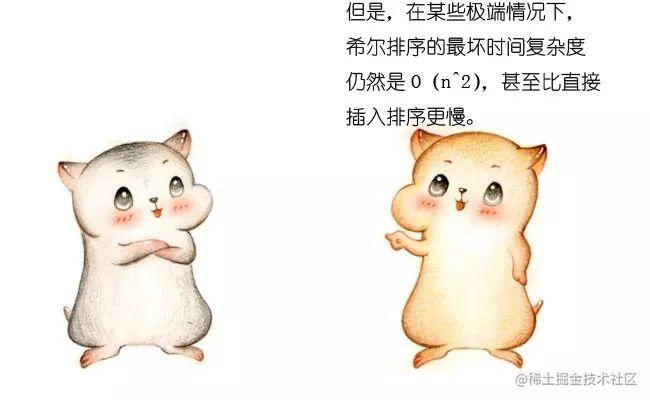Hibbard的增量序列如下：

1，3，7，15......

Sedgewick的增量序列如下：

1, 5, 19, 41, 109......—————END—————